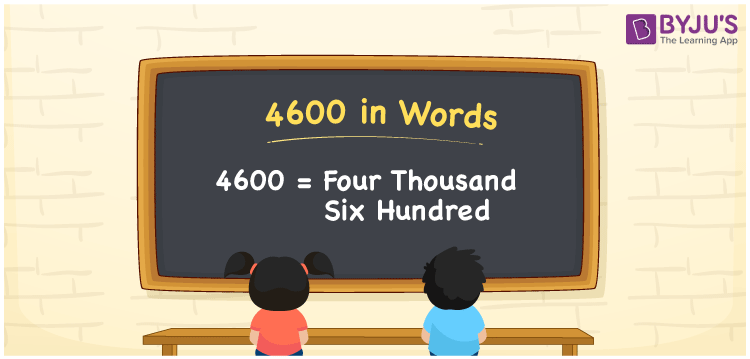# 4600 in words

4600 in words is written as Four Thousand Six Hundred. 4600 represents the count or value. The article on Counting Numbers can give you an idea about count or counting. The number 4600 is used in expressions that relate to money, distance, length and others. Let us consider an example for 4600. “On a 7 day car trip, we covered Four Thousand Six Hundred kilometers”. Another example, “Four Thousand Six Hundred grams is the same as 4.6 kilograms”.

 4600 in words Four Thousand Six Hundred Four Thousand Six Hundred in Numbers 4600

## 4600 in English Words## How to Write 4600 in Words?

We can convert 4600 to words using a place value chart. This can be done as follows. The number 4600 has 4 digits, so let’s make a chart that shows the place value up to 4 digits.

 Thousands Hundreds Tens Ones 4 6 0 0

Thus, we can write the expanded form as:

4 × Thousand + 6 × Hundred + 0 × Ten + 0 × One

= 4 × 1000 + 6 × 100 + 0 × 10 + 0 × 1

= 4600

= Four Thousand Six Hundred.

4600 is the natural number that is succeeded by 4599 and preceded by 4601.

4600 in words –Four Thousand Six Hundred.

Is 4600 an odd number? – No.

Is 4600 an even number? – Yes.

Is 4600 a perfect square number? – No.

Is 4600 a perfect cube number? – No.

Is 4600 a prime number? – No.

Is 4600 a composite number? – Yes.

## Solved Example

1. Write the number 4600 in expanded form

Solution: 4 × 1000 + 6 × 100 + 0 × 10 + 0 × 1.

We can write 4600 = 4000 + 600 + 0 + 0

= 4 × 1000 + 6 × 100 + 0 × 10 + 0 × 1.

## Frequently Asked Questions on 4600 in words

Q1

### How to write 4600 in words?

4600 in words is written as Four Thousand Six Hundred.
Q2

### State whether True or False. 4600 is divisible by 3?

False. 4600 is not divisible by 3.
Q3

### Is 4600 divisible by 10?

Yes. 4600 is divisible by 10.